Chapter 4 Class 10 Quadratic Equations

Class 10
Important Questions for Exam - Class 10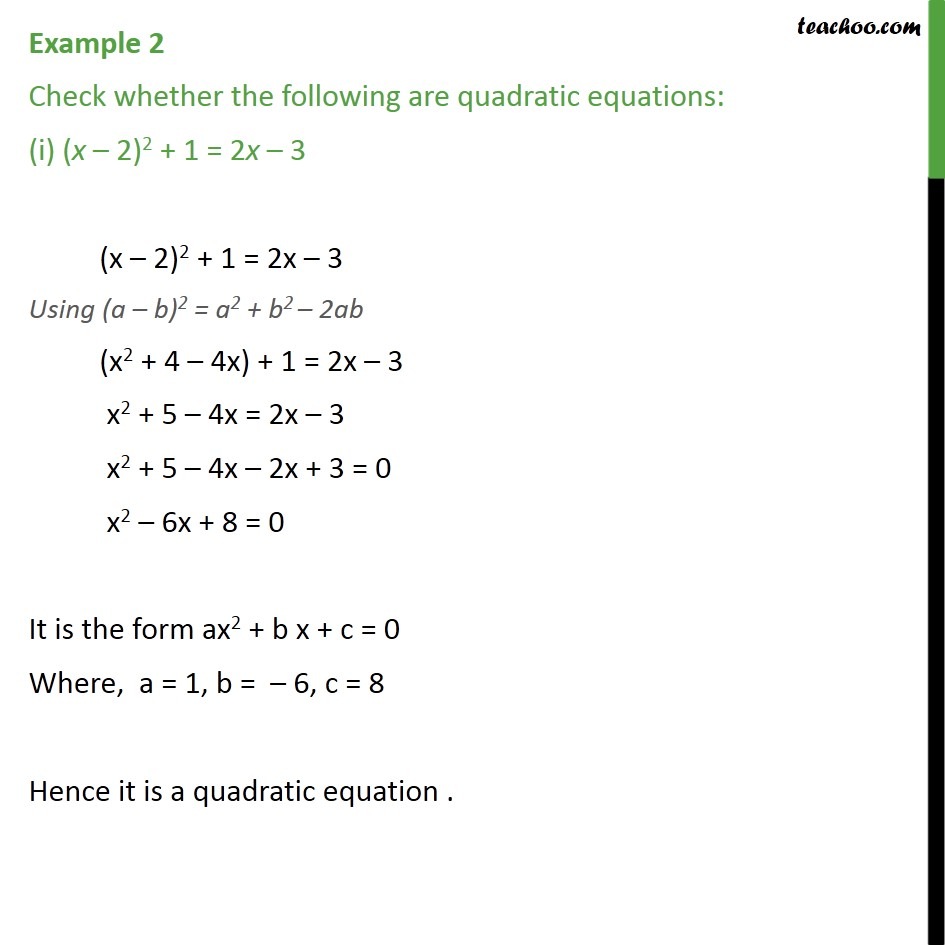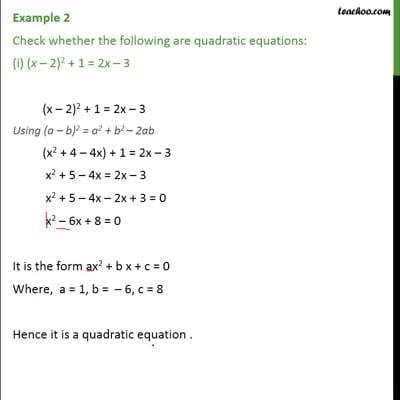This video is only available for Teachoo black users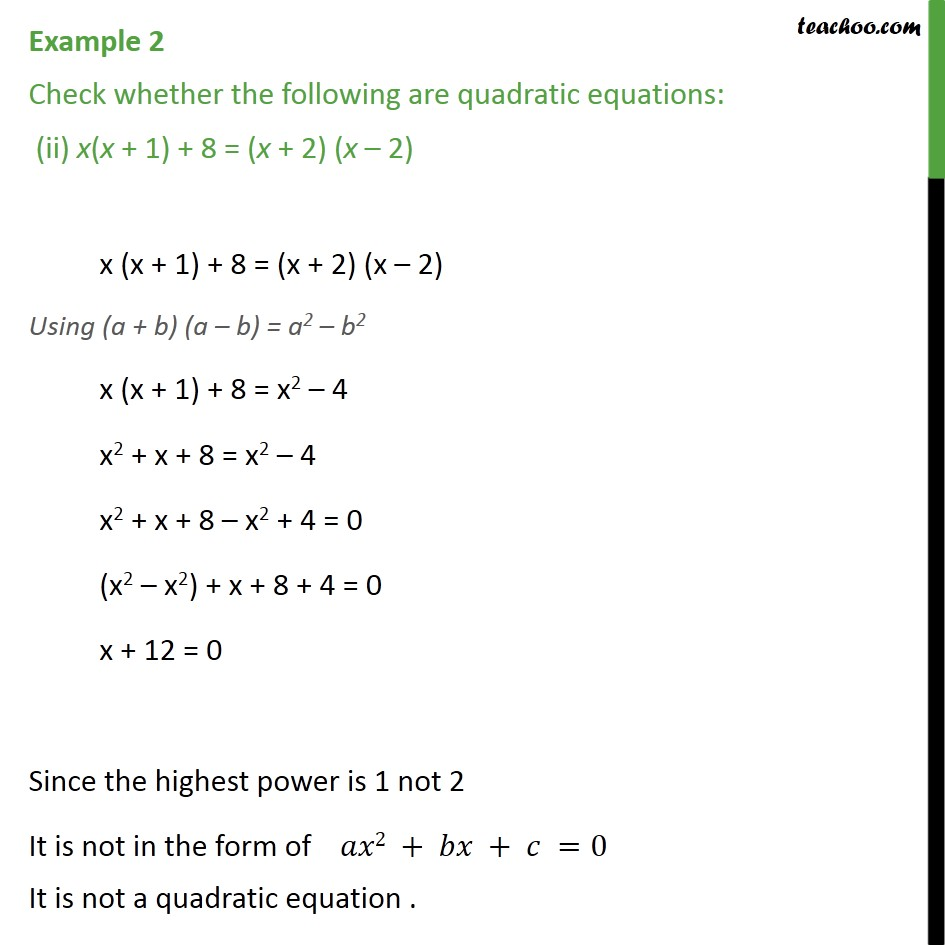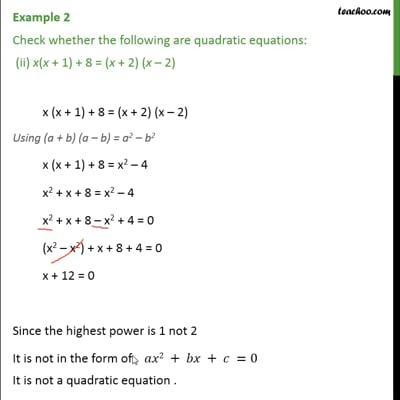This video is only available for Teachoo black users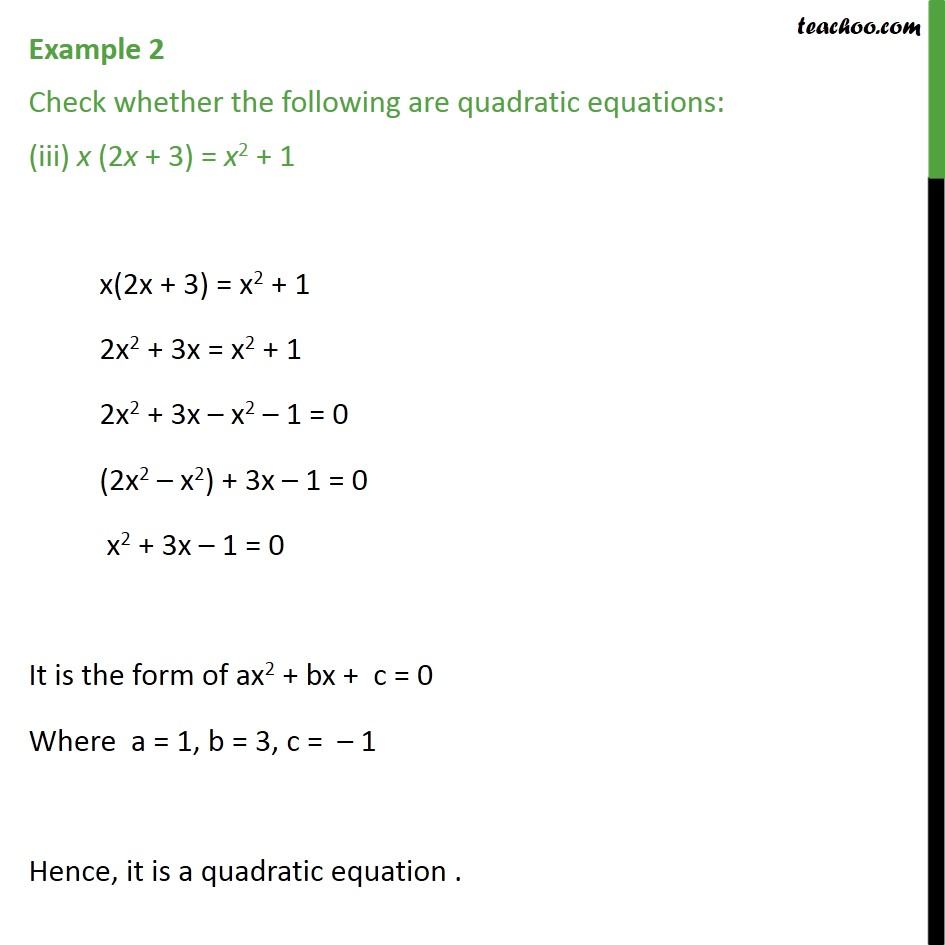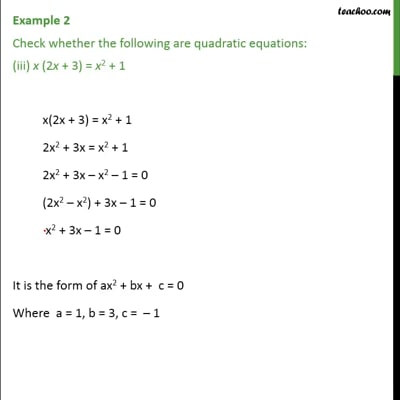This video is only available for Teachoo black users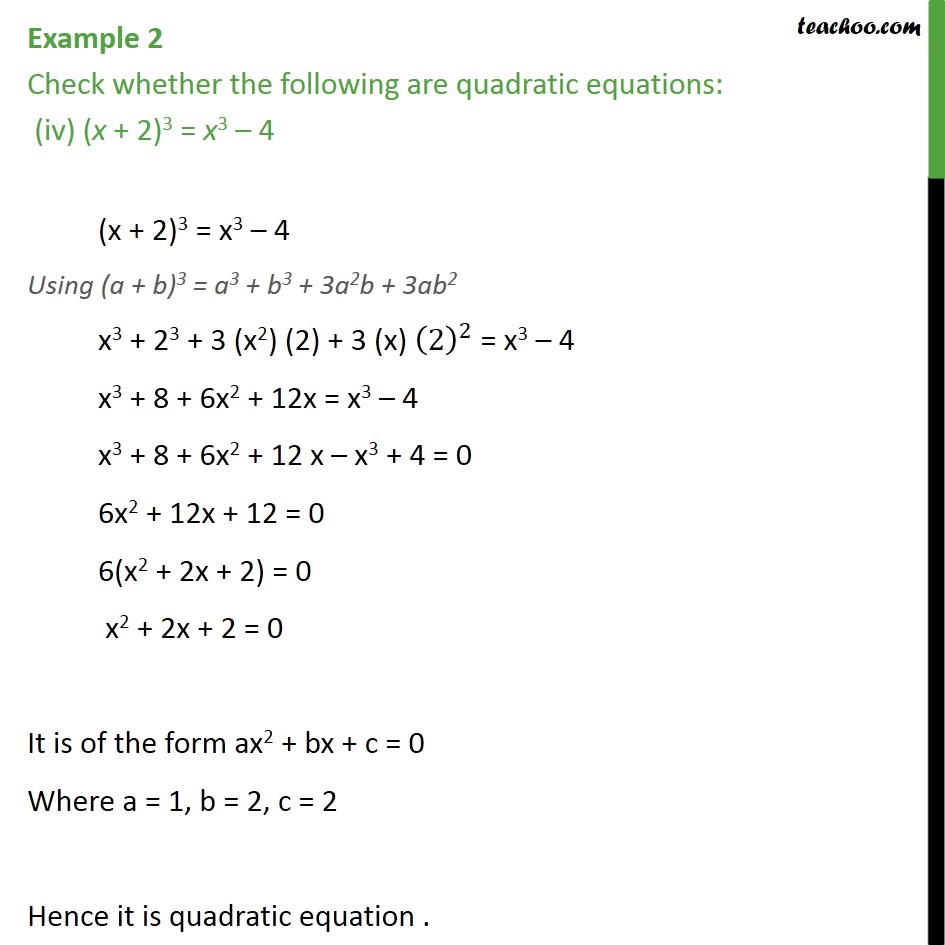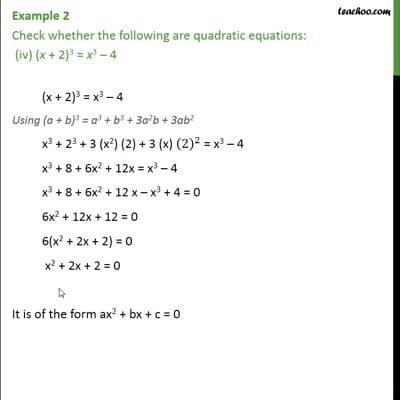This video is only available for Teachoo black users

### Transcript

Example 2 Check whether the following are quadratic equations: (i) (x 2)2 + 1 = 2x 3 (x 2)2 + 1 = 2x 3 Using (a b)2 = a2 + b2 2ab (x2 + 4 4x) + 1 = 2x 3 x2 + 5 4x = 2x 3 x2 + 5 4x 2x + 3 = 0 x2 6x + 8 = 0 It is the form ax2 + b x + c = 0 Where, a = 1, b = 6, c = 8 Hence it is a quadratic equation . Example 2 Check whether the following are quadratic equations: (ii) x(x + 1) + 8 = (x + 2) (x 2) x (x + 1) + 8 = (x + 2) (x 2) Using (a + b) (a b) = a2 b2 x (x + 1) + 8 = x2 4 x2 + x + 8 = x2 4 x2 + x + 8 x2 + 4 = 0 (x2 x2) + x + 8 + 4 = 0 x + 12 = 0 Since the highest power is 1 not 2 It is not in the form of 2 + + =0 It is not a quadratic equation . Example 2 Check whether the following are quadratic equations: (iii) x (2x + 3) = x2 + 1 x(2x + 3) = x2 + 1 2x2 + 3x = x2 + 1 2x2 + 3x x2 1 = 0 (2x2 x2) + 3x 1 = 0 x2 + 3x 1 = 0 It is the form of ax2 + bx + c = 0 Where a = 1, b = 3, c = 1 Hence, it is a quadratic equation . Example 2 Check whether the following are quadratic equations: (iv) (x + 2)3 = x3 4 (x + 2)3 = x3 4 Using (a + b)3 = a3 + b3 + 3a2b + 3ab2 x3 + 23 + 3 (x2) (2) + 3 (x) (2)^2 = x3 4 x3 + 8 + 6x2 + 12x = x3 4 x3 + 8 + 6x2 + 12 x x3 + 4 = 0 6x2 + 12x + 12 = 0 6(x2 + 2x + 2) = 0 x2 + 2x + 2 = 0 It is of the form ax2 + bx + c = 0 Where a = 1, b = 2, c = 2 Hence it is quadratic equation